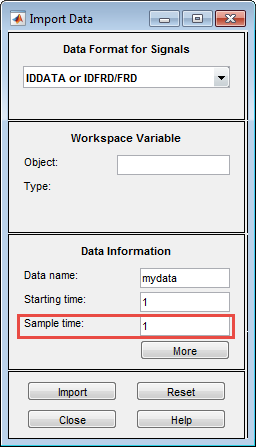## Specifying the Data Sample Time

When you import data into the app, you must specify the data sample time.

The sample time is the time between successive data samples in your experiment and must be the numerical time interval at which your data is sampled in any units. For example, enter `0.5` if your data was sampled every `0.5 s`, and enter `1` if your data was sampled every `1 s`.

You can also use the sample time as a flag to specify continuous-time data. When importing continuous-time frequency domain or frequency-response data, set the Sample time to `0`.

The sample time is used during model estimation. For time-domain data, the sample time is used together with the start time to calculate the sampling time instants. When you transform time-domain signals to frequency-domain signals (see the `fft` reference page), the Fourier transforms are computed as discrete Fourier transforms (DFTs) for this sample time. In addition, the sampling instants are used to set the horizontal axis on time plots.

Sample Time in the Import Data dialog box## SupportGet trial now